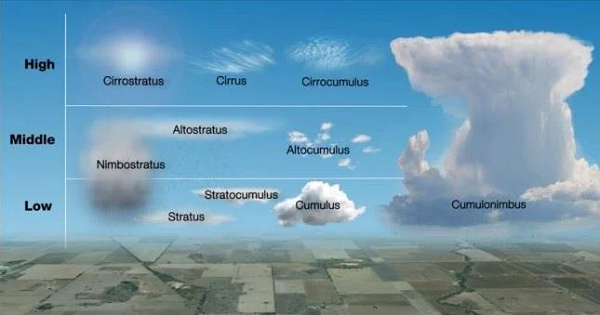# General Knowledge - Atmosphere

• The composition of the Earth’s atmosphere changes with the altitude.

• The major constituents of the Earth’s atmosphere are −

• Nitrogen – 78.09%

• Oxygen – 20.95%

• Argon – 0.93%

• Carbon Dioxide – 0.039% (remaining other gasses)

• The pressure exerted by the weight of air at a given point is known as “Atmospheric Pressure” or “Barometric Pressure.”

• With increasing altitude, the atmospheric pressure decreases.

• On an average, a column of air (which is normally one square centimeter in cross-section), measured at the sea level, has the weight of about 1.03 kg (about 10.1 N).

• The Average Air Pressure is about 14.70 pounds per square inch, (equivalent to 1,013.25 × 103 dynes per square centimeter or 1,013.25 millibars) at the sea level.

• A visible mass of condensed vapor floating above the general level of the ground is known as Cloud.

• Based on the altitude, cloud is classified as −

• High Altitude Cloud: For example, Cirrus, Cirrocumulus, & Cirrostratus.

• Middle Altitude Cloud: For example, Altostratus and Altocumulus.

• Low Altitude Cloud: Stratus, Stratocumulus, Cumulus, and Nimbostratus (it can be also seen in the middle altitude).

• Vertical: Cumulonimbus